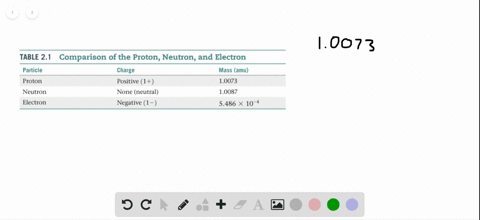Sign up for our free STEM online summer camps starting June 1st!View Summer Courses### It is common in mass spectrometry to assume that …

03:18Manhattan College

Need more help? Fill out this quick form to get professional live tutoring.

Get live tutoring
Problem 98

There are two different isotopes of bromine atoms. Under normal conditions, elemental bromine consists of Br_{2} \text molecules, and the mass of a Br $_{2}$ molecule is the sum of the masses of the two atoms in the molecule. The mass spectrum of Br_{2} consists of three peaks:(a) What is the origin of each peak (of what isotopes does each consist)? (b) What is the mass of each isotope? (c) Determine the average molecular mass of a Br_ molecule. (d) Determine the average atomic mass of a bromine atom. (e) Calculate the abundances of the two isotopes.

## Discussion

You must be signed in to discuss.

## Video Transcript

you guys were doing problem in idea of Chapter two chemistry Central science. So they're two different isotopes of Roman times under normal conditions. Elemental broom, inconsistent br, too and the mass of beer. Two molecules. It's on the mast to atoms in the molecule. The mass by Trump here to consist three peaks was the origin of each peak of what I steps. Each consists access, so each other. So we know that the but the weakest peak is our day peak with the smallest that's mass and speak with the largest mass. Those are going to be he having both our isotopes together, the other world to peak in the middle. That's going to be a composite of each isotope. So if we add if we ran this at the same 1 58 this may want t to We divide that by half, so we have B R 79 and B R B r a. T one. So that means that this is going that this peak is going to do to be our 79 and b r 79. This is going to be br Anyone be are 81 and this is gonna be B R 79 and B R a one one. Okay, so what is the mass of each? What is the mass of each isotope? More specific. Quickly. We are 79. That's gonna be 1 57.836 Me too, Because 78 0.91 ain't 8 a.m. U. And by someone, Katie. Well, 916 a m u. There's the massively just took determined the average molecular mass of a B R molecule. So we know the the size of each peak. So the way we are going to do that is going to be the molecular weight times the relative abundance. Now we're gonna find the average okay to the average molecular weight can be 1 59 point seven 90 a m. U. So they want term Is the average atomic mass of a bro mean Adam? And so we're just going. So we just need to So we're just going to to this? Yes, we have to do basically what we did in this equation. We have to wait them depending on their relative size, if size, or are simply we can just do this. So this is the average weight of the B R two molecules. So let me just to be us. Then we'll just divide this by two 790 over to. That's going to equal 79 point 895 AM you? Then we can calculate the relative abundance of each two of each of the isotopes. It does tough. So so it's going to be 79 point 918 x plus What. 80.916 times one minus X Because the abundance of the Because the abundance of the other isotope has to be the buns of the tourist shops have equal to one, and that's going to equal 17. 79 point 895 Come on. Oh, that 78. I need a 0.918 Excellent. 8.916 Money, eh? 0.9 six acts is equal to 79.895 It's a minus one, isn't it? 0.98 x that minus 1.21 Tow X is equal to 0.55 Is there a 0.5100 and one minus zero point 5100 one minus 0.49 for date night. Where is that means burrow means 74. So it started burning 79. We have 51.1% abundance us and burning 81 is equal to 48.9% abundance.